Published

# Wheel Rotation IOT Project

Ever park in a tight spot and forget which way your tires are pointed? Well fear no more! With this particle argon configuration.

BeginnerFull instructions provided302## Things used in this project

### Hardware componentsUltrasonic Sensor - HC-SR04 (Generic)
×2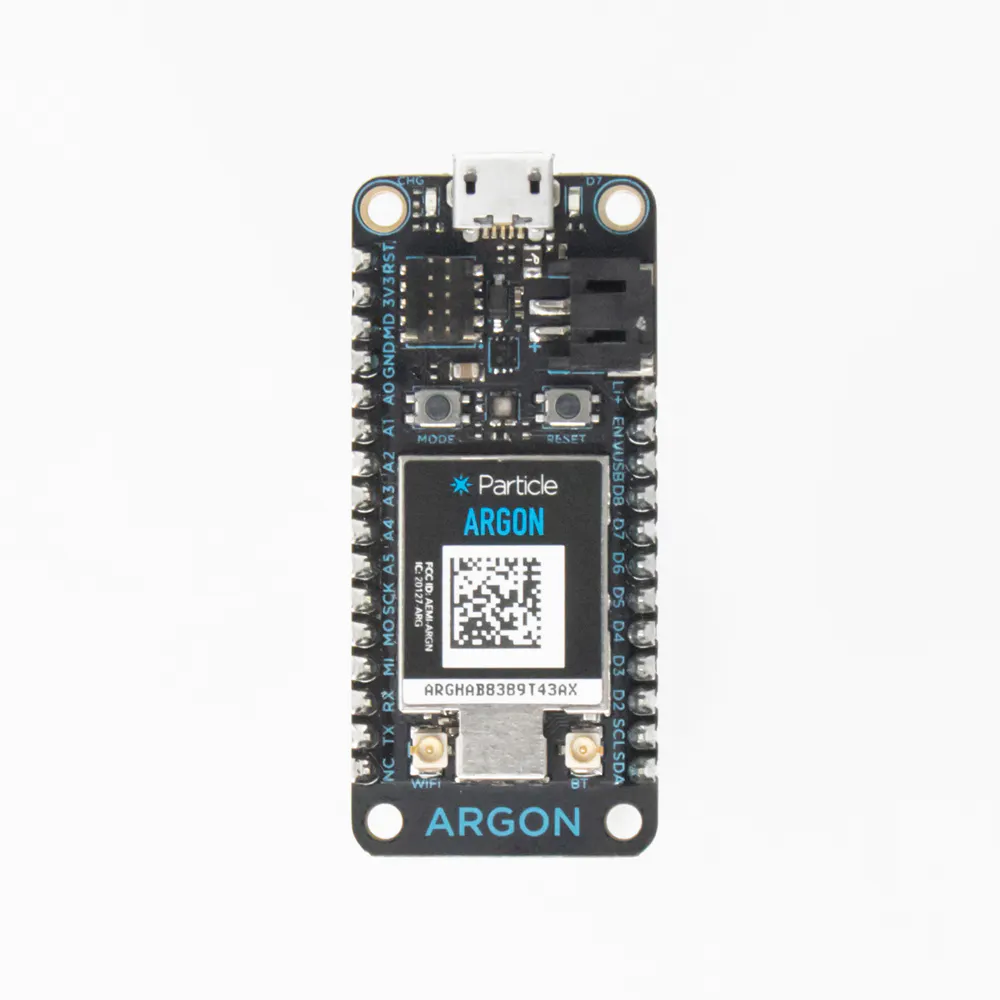Particle Argon
×2
 button
×1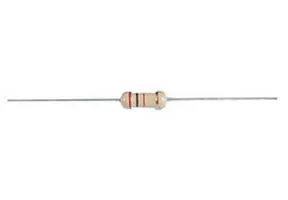Through Hole Resistor, 2.2 kohm
×1LED (generic)
×1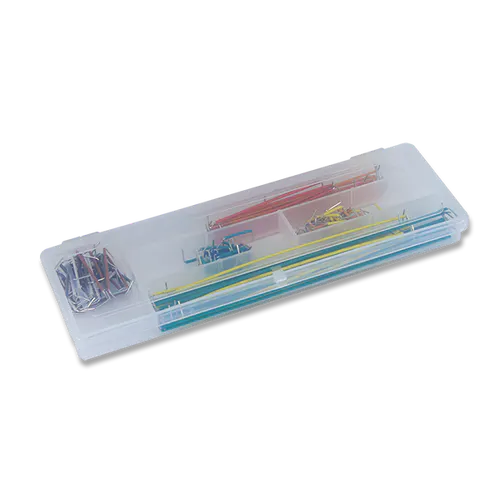×1

## Schematics

### Sensor Array

Wiring schematic for the sensor array. The 5V and ground in our scenario was from another board which could supply the 5V. The pins on the schematic MUST match that of the code to work properly, so if you decide to change any of the pin configurations on either side, you must change it on the other.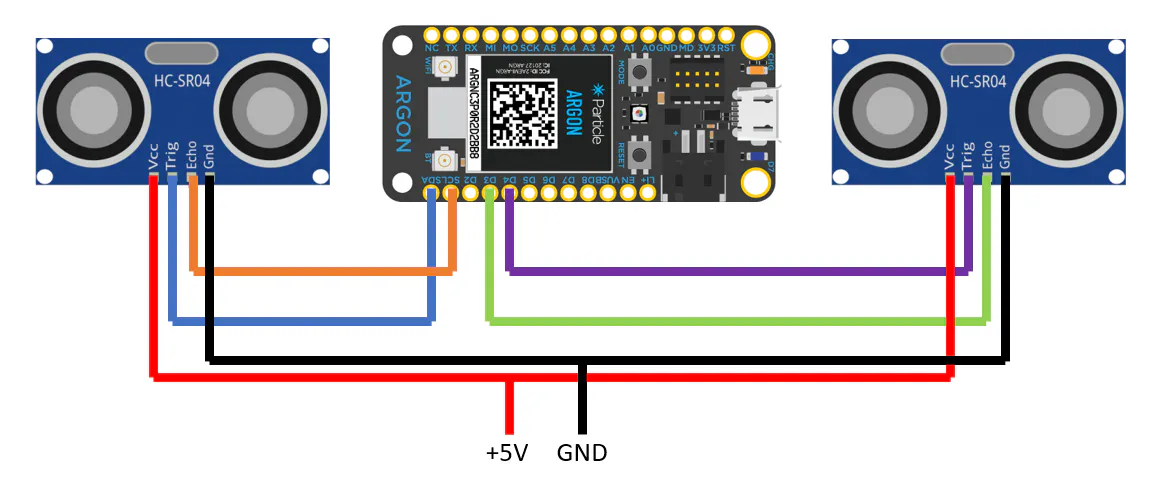### Button Argon

Wiring schematic for the button argon. Based on the voltage input, different resistor values may be required.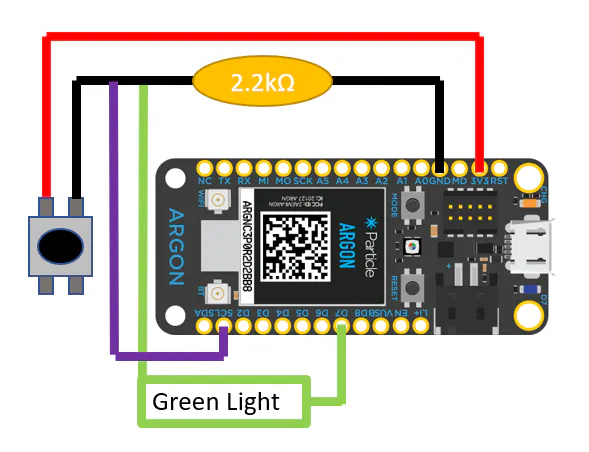## Code

### Sensor Array

C#
This code will take distance measurements for each argon run them through a few methods where a rough angle is calculated then sent to the other argon. The pins in particular do not matter as long as they are Digital pins. NOTE: the coding language is .ino, but for visual representation C# was chosen, as it was the closest to ino.
```//Ultrasonic Sensor Pins
const int pinEchoLeft = D1;
const int pinTrigLeft = D0;
const int pinEchoRight = D4;
const int pinTrigRight = D3;

//Variables Used For Calculations
double distLeft=0;
double distRight=0;
double angle=0;
long durationLeft;
long durationRight;
int lightOn;

void setup() {

//Setting Pins for their respective jobs
pinMode(pinTrigLeft,OUTPUT);
pinMode(pinEchoLeft,INPUT);
pinMode(pinTrigRight,OUTPUT);
pinMode(pinEchoRight,INPUT);

//Getting Variables to display on app
Particle.variable("Angle",angle);
Particle.variable("isOn",lightOn);
Serial.begin(9600);
}

void loop() {

//Calculates Left Sensor Data
digitalWrite(pinTrigLeft,LOW);
delayMicroseconds(2);
digitalWrite(pinTrigLeft,HIGH);
delayMicroseconds(10);
digitalWrite(pinTrigLeft,LOW);
durationLeft = pulseIn(pinEchoLeft,HIGH);
distLeft = durationLeft*0.034/2;

//Calculates Right Sensor Data
digitalWrite(pinTrigRight,LOW);
delayMicroseconds(2);
digitalWrite(pinTrigRight,HIGH);
delayMicroseconds(10);
digitalWrite(pinTrigRight,LOW);
durationRight = pulseIn(pinEchoRight,HIGH);
distRight = durationRight*0.034/2;

//This is The Variable we need
angle = calculateAngle(distLeft,distRight,11.4);

if (angle <10) {
lightOn = 1;
Particle.publish("ledOn",PRIVATE);
delay(300);
} else {
lightOn = 0;
Particle.publish("ledOff",PRIVATE);
delay(300);
}
delay(2000);
}

double calculateAngle(double left, double right, double width) {
if (right > left) {
right = right - left;
return arctan(right/width);
} else if (left > right) {
left = left - right;
return arctan(left/width);
}
}

double arctan(double input) {
//For some reason arctan and atan did not exist so we will use approximation
if ((input > 0)&&(input <0.08748864)) {
return 0;
} else if ((input > 0.08748864)&&(input < 0.176326981)) {
return 5;
} else if ((input > 0.176326981)&&(input < 0.2679491912)) {
return 10;
} else if ((input > 0.2679491912)&&(input < 0.363970234)) {
return 15;
} else if ((input > 0.363970234)&&(input < 0.466307658)) {
return 20;
} else if ((input > 0.466307658)&&(input < 0.577350269)) {
return 25;
} else if ((input > 0.577350269)&&(input < 0.700207538)) {
return 30;
} else if ((input > 0.700207538)&&(input < 0.839099631)) {
return 35;
} else {
return 40;
}
}
```

### Button Argon

C#
This code receives data from the other argon and dependent on the packet it receives will turn the light indicator on or off. Note if no light is plugged into D7, the on board light will also turn on.
```int pinCheck = D7;
int pinIn = D1;
bool val;

void setup() {
pinMode(pinCheck, OUTPUT);
pinMode(pinIn, INPUT);

Particle.variable("On/Off", val);
Particle.subscribe("ledOn",subToArgon,MY_DEVICES);
Particle.subscribe("ledOff",nothing,MY_DEVICES);
}

void loop(){
val = true;
} else {
val = false;
digitalWrite(pinCheck,LOW);
}

}

void subToArgon(const char *event, const char *data) {
if (val==true) {
digitalWrite(D7,HIGH);
delay(500);
digitalWrite(D7,LOW);
delay(500);
digitalWrite(pinCheck,HIGH);
}
}
void nothing(const char *event, const char *data) {
digitalWrite(pinCheck,LOW);

}
```

## Credits

### Sean McClanahan

1 project • 0 followers

### Grant Sonafrank

1 project • 0 followers